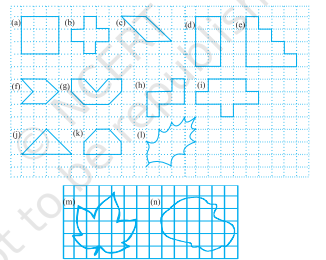# Find the areas of the following figures by counting square:"

To do:

We have to find the areas of the given figures by counting squares.

Solution:

(a) The number of fully filled squares in the given figure is 9 squares

Therefore,

The area of the given figure is $9$ square units.

(b) The number of fully filled squares in the given figure is 5 squares

Therefore,

The area of the given figure is $5$ square units.

(c) The number of fully filled squares in the given figure is 2 squares and there are 4 half-filled squares also,

Therefore,

The area of the given figure $=2+4\times\frac{1}{2}$

$=2+2$

$=4$ square units.

(d) The number of fully filled squares in the given figure is 8 squares

Therefore,

The area of the given figure is $8$ square units.

(e) The number of fully filled squares in the given figure is 10 squares

Therefore,

The area of the given figure is $10$ square units.

(f) The number of fully filled squares in the given figure is 2 squares and there are 4 half-filled squares also,

Therefore,

The area of the given figure $=2+4\times\frac{1}{2}$

$=2+2$

$=4$ square units.

(g) The number of fully filled squares in the given figure is 4 squares and there are 4 half filled squares also,

Therefore,

The area of the given figure $=4+4\times\frac{1}{2}$

$=4+2$

$=6$ square units.

(h) The number of fully filled squares in the given figure is 5 squares

Therefore,

The area of the given figure is $5$ square units.

(i) The number of fully filled squares in the given figure is 9 squares

Therefore,

The area of the given figure is $9$ square units.

(j) The number of fully filled squares in the given figure is 2 squares and there are 4 half-filled squares also,

Therefore,

The area of the given figure $=2+4\times\frac{1}{2}$

$=2+2$

$=4$ square units.

(k) The number of fully filled squares in the given figure is 2 squares and there are 4 half-filled squares also,

Therefore,

The area of the given figure $=4+2\times\frac{1}{2}$

$=4+1$

$=5$ square units.

(l)  From the given figure, we observe,

 Covered Area Number Area  (square units) Fully filled squares 2 2 Half filled squares 0 0 More than half-filled squares 6 6 Less than half-filled squares 6 0

Therefore,

The total area covered by squares $=2+6$

$= 8$ square units.

(m)  From the given figure, we observe,

 Covered Area Number Area  (square units) Fully filled squares 5 5 Half filled squares 0 0 More than half-filled squares 9 9 Less than half-filled squares 12 0

Therefore,

The total area covered by squares $=5+9$

$= 14$ square units.

(n) From the given figure, we observe,

 Covered Area Number Area  (square units) Fully filled squares 8 8 Half filled squares 0 0 More than half-filled squares 10 10 Less than half-filled squares 9 0

Therefore,

The total area covered by squares $=8+10$

$= 18$ square units.